# Cuboid + fundamentals of Physics - examples

A cuboid is a three-dimensional shape with a length, width, and a height. A cuboid is a rectangular Prism. The cuboid shape has six sides called faces. Each face of a cuboid is a rectangle, and all of a cuboid's corners (called vertices) are 90-degree angles. Opposite faces are parallel. A cuboid has the shape of a rectangular box.

1. ForcesIn point O acts three orthogonal forces: F1 = 20 N, F2 = 7 N and F3 = 19 N. Determine the resultant of F and the angles between F and forces F1, F2 and F3.
2. IcerinkRectangular rink with dimensions of 68.7 m and 561 dm must be covered with a layer of ice 4.2 cm thick. How many liters of water is necessary for the formation of ice when the volume of ice is 9.7% greater than the volume of water.
3. Cuboid 5Calculate the mass of the cuboid with dimensions of 12 cm; 0.8 dm and 100 mm made from spruce wood (density = 550 kg/m3).
4. Cuprum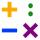From 1600 mm long sheet of copper plate 2 mm thickness we have separated over the whole length the belt weighing 6000 g. Calculate belt width if 1 dm3 copper weighs 8.9 kg.
5. Stone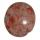When Peter threw stone in a box of water he discovered that the water level has risen by 9 cm. The box has a cuboid shape, the bottom has dimensions of 24 cm and 14 cm, height is 50 cm. What volume has a stone?
6. Ice and water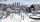We want to cover rectangular rink with dimensions of 55 m and 25 m with 4cm thick layer of ice. How many liters of water we need if after freezing water increases its volume by 10%?
7. Fe vs. H2O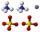The volume of what the body of the same weight is greater - iron or water?
8. Wood cuboidWhat is the weight of the wood cuboid 15 cm, 20 cm, 3 m if 1 m3 wood weighs 800 kg?
9. FreezerThe freezer has the shape of a cuboid with internal dimensions of 12 cm, 10 cm, 30 cm. A layer of ice of 23 mm thick was formed on the inner walls (and on the opening) of the freezer. How many liters of water will drain if we dispose the freezer?
10. Wood lumberWooden lumber is 4 m long and has a cross section square with side 15 cm. Calculate: a) the volume of lumber b) the weight of the lumber if 1 m3 weighs 790 kg
11. Water level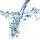To cuoid shaped poll, bottom size 2m and 3.5m; flows water at a rate of 50 liters per minute. How long will take to water reach level 50 cm?
12. IcebergWhat is the surface area of 50 cm iceberg (in the shape of a cuboid) that can carry a man with luggage with a total weight of 120 kg?

We apologize, but in this category are not a lot of examples.
Do you have an interesting mathematical example that you can't solve it? Enter it, and we can try to solve it.

To this e-mail address, we will reply solution; solved examples are also published here. Please enter e-mail correctly and check whether you don't have a full mailbox.